# Guide to spatially-structured networks¶

The NEST simulator 1 provides a convenient interface for creating neurons placed in space and connecting those neurons with probabilities and properties depending on the relative placement of neurons. This permits the creation of complex networks with spatial structure.

This user manual provides an introduction to the functionalities provided for defining spatial networks in NEST. It is based exclusively on the PyNEST, the Python interface to NEST. NEST users using the SLI interface should be able to map instructions to corresponding SLI code. This manual is not meant as a comprehensive reference manual. Please consult the online documentation in PyNEST for details; where appropriate, that documentation also points to relevant SLI documentation.

This manual describes the spatial functionalities included with NEST 3.0.

In the next section of this manual, we introduce spatially distributed nodes. In Chapter 3 we then describe how to connect spatial nodes with each other, before discussing in Chapter 4 how you can inspect and visualize spatial networks. Chapter 5 deals with creating connection boundaries using parameters, and the more advanced topic of extending the functionalities with custom masks provided by C++ classes in an extension module.

You will find the Python scripts used in the examples in this manual in the NEST source code directory under doc/guides/spatial/user_manual_scripts.

## Limitations and Disclaimer¶

Undocumented features

There may be a number of undocumented features, which you may discover by browsing the code. These features are highly experimental and should not be used for simulations, as they have not been validated.

## Structurally distributed nodes¶

Neuronal networks can have an organized spatial distribution, which we call layers. Layers in NEST 3.0 are NodeCollections with spatial metadata. We will first illustrate how to place elements in simple grid-like layers, where each element is a single model neuron, before describing how the elements can be placed freely in space.

We will illustrate the definition and use of spatially distributed NodeCollections using examples.

NEST distinguishes between two classes of layers:

grid-based layers

in which each element is placed at a location in a regular grid;

free layers

in which elements can be placed arbitrarily in the plane.

Grid-based layers allow for more efficient connection-generation under certain circumstances.

### Grid-based NodeCollections¶

#### A very simple example¶

We create a first, grid-based simple NodeCollection with the following command:

layer = nest.Create('iaf_psc_alpha',
positions=nest.spatial.grid(shape=[5, 5]))Figure 7 Simple grid-based NodeCollection centered about the origin. Blue circles mark the elements, the thin square the extent of the layer. Row and column indices are shown in the right and top margins, respectively.

The layer is shown in Figure 7. Note the following properties:

• We are using the standard Create function, but in addition to model type, we are also passing a nest.spatial.grid object as the positions argument.

• The layer has five rows and five columns, as given by shape=[columns, rows]. Note that a more intuitive way of thinking of shape is to think of it as given by shape=[num_x, num_y].

• The center of the NodeCollection is at the origin of the coordinate system, $$(0,0)$$.

• The extent or size of the layer is $$1\times 1$$. This is the default size for grid-based layers. The extent is marked by the thin square in Figure 7.

• The grid spacing of the layer is

$\begin{split}\begin{split} dx &= \frac{\text{x-extent}}{\text{number of columns}} \\ dy &= \frac{\text{y-extent}}{\text{number of rows}} \end{split}\end{split}$

In the layer shown, we have $$dx=dy=0.2$$, but the grid spacing may differ in x- and y-direction.

• Layer elements are spaced by the grid spacing and are arranged symmetrically about the center.

• The outermost elements are placed $$dx/2$$ and $$dy/2$$ from the borders of the extent.

• Element positions in the coordinate system are given by $$(x,y)$$ pairs. The coordinate system follows that standard mathematical convention that the $$x$$-axis runs from left to right and the $$y$$-axis from bottom to top.

• Each element of a grid-based NodeCollection has a row- and column-index in addition to its $$(x,y)$$-coordinates. Indices are shown in the top and right margin of Figure 7. Note that row-indices follow matrix convention, i.e., run from top to bottom. Following pythonic conventions, indices run from 0.

#### Setting the extent¶

Grid-based layers have a default extent of $$1\times 1$$. You can specify a different extent of a layer, i.e., its size in $$x$$- and $$y$$-direction by passing the extent argument to nest.spatial.grid():

layer = nest.Create('iaf_psc_alpha',
positions=nest.spatial.grid(
shape=[5, 5],
extent=[2.0, 0.5]))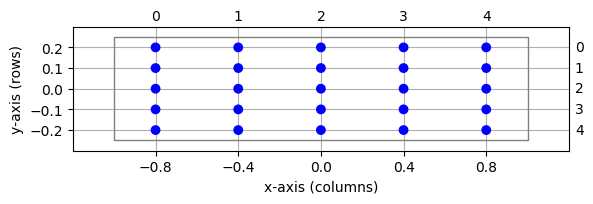Figure 8 Same layer as in Figure 7, but with different extent.

The resulting NodeCollection is shown in Figure 8. The extent is always a two-element list of floats. In this example, we have grid spacings $$dx=0.4$$ and $$dy=0.1$$. Changing the extent does not affect grid indices.

The size of extent in $$x$$- and $$y$$-directions should be numbers that can be expressed exactly as binary fractions. This is automatically ensured for integer values. Otherwise, under rare circumstances, subtle rounding errors may occur and trigger an assertion, thus stopping NEST.

#### Setting the center¶

Layers are centered about the origin $$(0,0)$$ by default. This can be changed by passing the center argument to nest.spatial.grid(). The following code creates layers centered about $$(0,0)$$, $$(-1,1)$$, and $$(1.5,0.5)$$, respectively:

layer1 = nest.Create('iaf_psc_alpha',
positions=nest.spatial.grid(shape=[5, 5]))
layer2 = nest.Create('iaf_psc_alpha',
positions=nest.spatial.grid(
shape=[5, 5],
center=[-1., 1.]))
layer3 = nest.Create('iaf_psc_alpha',
positions=nest.spatial.grid(
shape=[5, 5],
center=[1.5, 0.5]))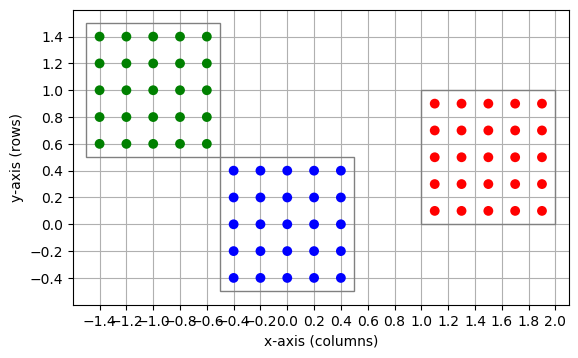Figure 9 Three layers centered, respectively, about $$(0,0)$$ (blue), $$(-1,-1)$$ (green), and $$(1.5,0.5)$$ (red).

The center is given as a two-element list of floats. Changing the center does not affect grid indices: For each of the three layers in

Figure 9, grid indices run from 0 to 4 through columns and

rows, respectively, even though elements in these three layers have different positions in the global coordinate system.

The center coordinates should be numbers that can be expressed exactly as binary fractions. For more information, see Sec. 2.1.2.

#### Constructing a layer: an example¶

To see how to construct a layer, consider the following example:

• a layer with $$n_y$$ rows and $$n_x$$ columns;

• spacing between nodes is $$d$$ in $$x$$- and $$y$$-directions;

• the left edge of the extent shall be at $$x=0$$;

• the extent shall be centered about $$y=0$$.

From Eq. dx_dy_extent, we see that the extent of the NodeCollection must be $$(n_x d, n_y d)$$. We now need to find the coordinates $$(c_x, c_y)$$ of the center of the layer. To place the left edge of the extent at $$x=0$$, we must place the center of the layer at $$c_x=n_x d / 2$$ along the $$x$$-axis, i.e., half the extent width to the right of $$x=0$$. Since the layer is to be centered about $$y=0$$, we have $$c_y=0$$. Thus, the center coordinates are $$(n_x d/2, 0)$$. The layer is created with the following code and shown in Figure 10:

nx, ny = 5, 3
d = 0.1
layer = nest.Create('iaf_psc_alpha',
positions=nest.spatial.grid(
shape=[nx, ny],
extent=[nx * d, ny * d],
center=[nx * d / 2., 0.]))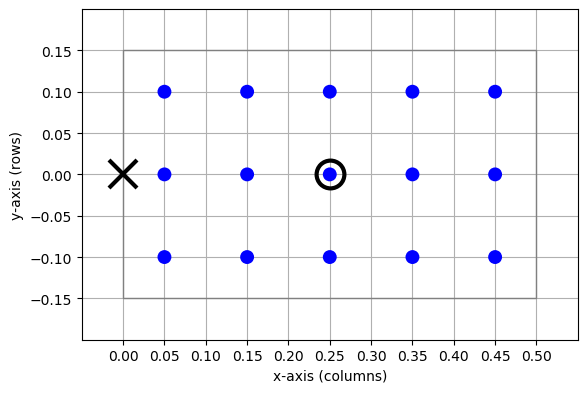Figure 10 NodeCollection with $$n_x=5$$ columns and $$n_y=3$$ rows, spacing $$d=0.1$$ and the left edge of the extent at $$x=0$$, centered about the $$y$$-axis. The cross marks the point on the extent placed at the origin $$(0,0)$$, the circle the center of the layer.

### Free layers¶

Free layers do not restrict node positions to a grid, but allow free placement within the extent. To this end, the user can specify the positions of all nodes explicitly, or pass a random distribution parameter to nest.spatial.free(). The following code creates a NodeCollection of 50 iaf_psc_alpha neurons uniformly distributed in a layer with extent $$1\times 1$$, i.e., spanning the square $$[-0.5,0.5]\times[-0.5,0.5]$$:

pos = nest.spatial.free(pos=nest.random.uniform(min=-0.5, max=0.5),
num_dimensions=2)
layer = nest.Create('iaf_psc_alpha', 50,
positions=pos)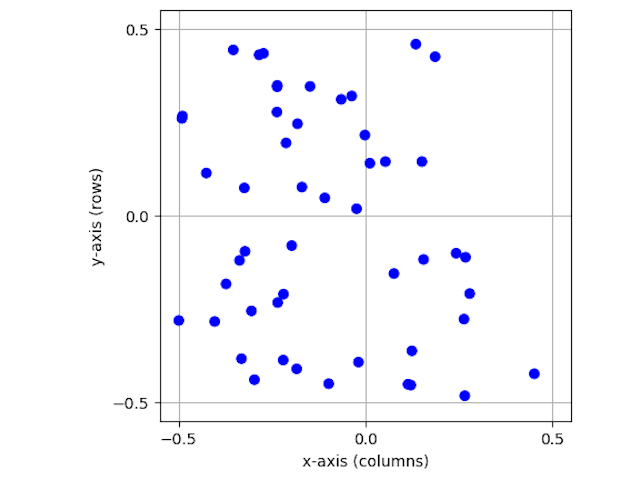Figure 11 A free layer with 50 elements uniformly distributed in an extent of size $$1\times 1$$.

Note the following points:

• For free layers, element positions are specified by the nest.spatial.free object.

• The pos entry must either be a Python list (or tuple) of element coordinates, i.e., of two-element tuples of floats giving the ($$x$$, $$y$$)-coordinates of the elements, or a Parameter object.

• When using a parameter object for the positions, the number of dimensions have to be specified by the num_dimensions variable. num_dimensions can either be 2 or 3.

• When using a parameter object you also need to specify how many elements you want to create by specifying 'n' in the Create call. This is not the case when you pass a list to the nest.spatial.free object.

• The extent is automatically set when using nest.spatial.free, however, it is still possible to set the extent yourself by passing the extent variable to the object.

• All element positions must be within the layer’s extent. Elements may be placed on the perimeter of the extent as long as no periodic boundary conditions are used; see Sec. 2.4.

To create a spatially distributed NodeCollection from a list, do the following:

pos = nest.spatial.free(pos=[[-0.5, -0.5], [-0.25, -0.25], [0.75, 0.75]])
layer = nest.Create('iaf_psc_alpha', positions=pos)Figure 12 A free layer with 3 elements freely distributed space. The extent is given by the gray lines.

Note that when using a list to specify the positions, neither 'n' nor num_dimenstions are specified. Furthermore, the extent is calculated from the node positions, and is here $$1.45\times 1.45$$. The extent could also be set by passing the extent argument.

### 3D layers¶

Although the term “layer” suggests a 2-dimensional structure, the layers in NEST may in fact be 3-dimensional. The example from the previous section may be easily extended by updating number of dimensions for the positions:

pos = nest.spatial.free(nest.random.uniform(min=-0.5, max=0.5),
num_dimensions=3)
layer = nest.Create('iaf_psc_alpha', 200, positions=pos)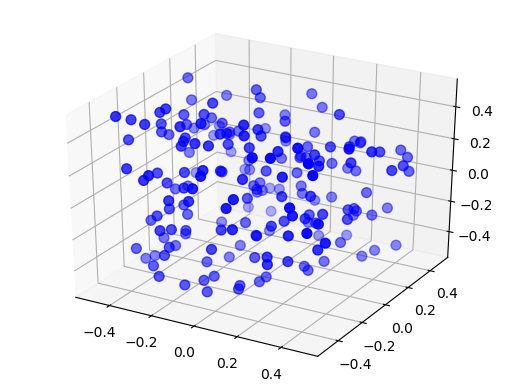Figure 13 A free 3D layer with 200 elements uniformly distributed in an extent of size $$1\times 1\times 1$$.

Again it is also possible to specify a list of list to create nodes in a 3-dimensional space. Another possibility is to create a 3D grid-layer, with 3 elements passed to the shape argument, shape=[nx, ny, nz]:

pos = nest.spatial.grid(shape=[4, 5, 6])
layer = nest.Create('iaf_psc_alpha', positions=pos)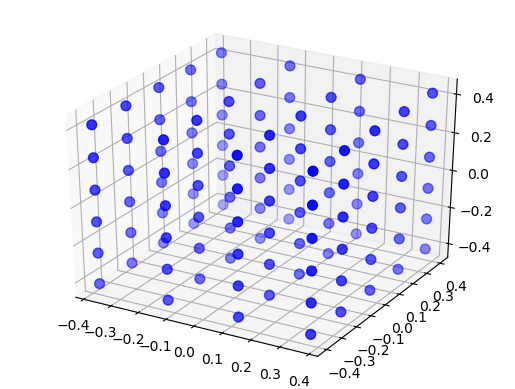Figure 14 A grid 3D NodeCollection with 120 elements distributed on a grid with 4 elements in the x-direction, 5 elements in the y-direction and 6 elements in the z-direction, with an extent of size $$1\times 1\times 1$$.

### Periodic boundary conditions¶

Simulations usually model systems much smaller than the biological networks we want to study. One problem this entails is that a significant proportion of neurons in a model network is close to the edges of the network with fewer neighbors than nodes properly inside the network. In the $$5\times 5$$-layer in Figure 7 for instance., 16 out of 25 nodes form the border of the layer.

One common approach to reducing the effect of boundaries on simulations is to introduce periodic boundary conditions, so that the rightmost elements on a grid are considered nearest neighbors to the leftmost elements, and the topmost to the bottommost. The flat layer becomes the surface of a torus. Figure 15 illustrates this for a one-dimensional layer, which turns from a line to a ring upon introduction of periodic boundary conditions.

You specify periodic boundary conditions for a NodeCollection using the entry edge_wrap:

layer = nest.Create('iaf_psc_alpha',
positions=nest.spatial.grid(
shape=[5, 1],
extent=[5., 1.],
edge_wrap=True))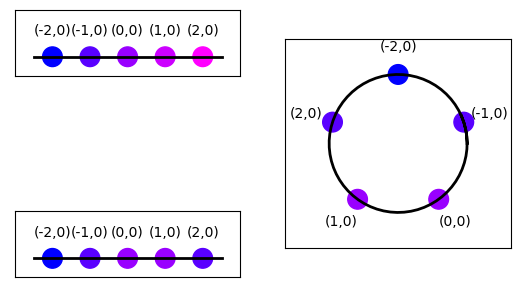Figure 15 Top left: Layer with single row and five columns without periodic boundary conditions. Numbers above elements show element coordinates. Colors shifting from blue to magenta mark increasing distance from the element at $$(-2,0)$$. Bottom left: Same layer, but with periodic boundary conditions. Note that the element at $$(2,0)$$ now is a nearest neighbor to the element at $$(-2,0)$$. Right: Layer with periodic boundary condition arranged on a circle to illustrate neighborhood relationships.

Note that the longest possible distance between two elements in a layer without periodic boundary conditions is

$\sqrt{x_{\text{ext}}^2 + y_{\text{ext}}^2}$

but only

$\left.\sqrt{x_{\text{ext}}^2 + y_{\text{ext}}^2}\right/ 2$

for a layer with periodic boundary conditions; $$x_{\text{ext}}$$ and $$y_{\text{ext}}$$ are the components of the extent size.

We will discuss the consequences of periodic boundary conditions more in Chapter 3.

### Layers as NodeCollection¶

From the perspective of NEST, a layer is a special type of NodeCollection. From the user perspective, the following points may be of interest:

• The NodeCollection has a spatial property describing the spatial properties of the NodeCollection (l is the layer created at the beginning of this guide):

print(layer.spatial)


The spatial property is read-only; changing any value will not change properties of the spatially distributed NodeCollection.

• NEST sees the elements of the layer in the same way as the elements of any other NodeCollection. NodeCollections created as layers can therefore be used in the same ways as any standard NodeCollection. However, operations requiring a NodeCollection with spatial data (e.g. Connect with spatial dependence, or visualization of layers) can only be used on NodeCollections created with spatial distribution.

nest.PrintNodes()


## Connections¶

The most important feature of the spatially-structured networks is the ability to create connections between NodeCollections with quite some flexibility. In this chapter, we will illustrate how to specify and create connections. All connections are created using the Connect function.

### Basic principles¶

#### Terminology¶

We begin by introducing important terminology:

Connection

In the context of connections between the elements of NodeCollections with spatial distributions, we often call the set of all connections between pairs of network nodes created by a single call to Connect a connection.

Connection dictionary

A dictionary specifying the properties of a connection between two NodeCollections in a call to Connect.

Source

The source of a single connection is the node sending signals (usually spikes). In a projection, the source layer is the layer from which source nodes are chosen.

Target

The target of a single connection is the node receiving signals (usually spikes). In a projection, the target layer is the layer from which target nodes are chosen.

Driver

When connecting two layers, the driver layer is the one in which each node is considered in turn.

Pool
When connecting two layers, the pool layer is the one from which nodes are chosen for each node in the driver layer. I.e., we have

Connection parameters

Driver

Pool

rule='pairwise_bernoulli'

source layer

target layer

rule='fixed_outdegree'

source layer

target layer

rule='pairwise_bernoulli' and use_on_source=True

target layer

source layer

rule='fixed_indegree'

target layer

source layer

Displacement

The displacement between a driver and a pool node is the shortest vector connecting the driver to the pool node, taking boundary conditions into account.

Distance

The distance between a driver and a pool node is the length of their displacement.

Mask

The mask defines which pool nodes are at all considered as potential targets for each driver node. See Sec. 3.3 for details.

Connection probability or p

The connection probability, specified as p in the connection specifications, is either a value, or a parameter which specifies the probability for creating a connection between a driver and a pool node. The default probability is $$1$$, i.e., connections are created with certainty. See Sec. 3.4 for details.

Autapse

An autapse is a synapse (connection) from a node onto itself. Autapses are permitted by default, but can be disabled by adding 'allow_autapses': False to the connection dictionary.

Multapse

Node A is connected to node B by a multapse if there are synapses (connections) from A to B. Multapses are permitted by default, but can be disabled by adding 'allow_multapses': False to the connection dictionary.

### Connecting spatially distributed nodes¶

As with “normal” NodeCollections, connections between spatially distributed nodes are created by calling Connect. However, having spatial information about the nodes makes position-based options available, and so in addition to the usual connection schemes there exists additional connection parameters for spatially distributed NodeCollections.

In many cases when connecting spatially distributed NodeCollections, a mask will be specified. Mask specifications are described in Sec. 3.3. Only neurons within the mask are considered as potential sources or targets. If no mask is given, all neurons in the respective NodeCollection are considered sources or targets.

Here is a simple example, cf. Figure 16

spatial_nodes = nest.Create('iaf_psc_alpha',
positions=nest.spatial.grid(shape=[11, 11], extent=[11., 11.]))
conndict = {'rule': 'pairwise_bernoulli',
'p': 1.0,
'mask': {'rectangular': {'lower_left': [-2., -1.],
'upper_right': [2., 1.]}}}
nest.Connect(spatial_nodes, spatial_nodes, conndict)Figure 16 Left: Minimal connection example from a layer onto itself using a rectangular mask shown as red for the node at $$(0,0)$$ (marked light red). The targets of this node are marked with red dots. The targets for the node at $$(4,5)$$ are marked with yellow dots. This node has fewer targets since it is at the corner and many potential targets are beyond the layer. Right: The effect of periodic boundary conditions is seen here. Source and target layer and connection dictionary were identical, except that periodic boundary conditions were used. The node at $$(4,5)$$ now has 15 targets, too, but they are spread across the corners of the layer. If we wrapped the layer to a torus, they would form a $$5\times 3$$ rectangle centered on the node at $$(4,5)$$.

In this example, layer l is both source and target layer. For each node in the NodeCollection we choose targets according to the rectangular mask centered about each source node. Since the connection probability is 1.0, we connect to all nodes within the mask. Note the effect of normal and periodic boundary conditions on the connections created for different nodes in the NodeCollection, as illustrated in Figure 16.

### Mapping source and target layers¶

The application of masks and other functions depending on the distance or even the displacement between nodes in the source and target layers requires a mapping of coordinate systems between source and target layers. NEST applies the following coordinate mapping rules:

1. All layers have two-dimensional Euclidean coordinate systems.

2. No scaling or coordinate transformation can be applied between NodeCollections with spatial distribution.

3. The displacement $$d(D,P)$$ from node $$D$$ in the driver layer to node $$P$$ in the pool layer is measured by first mapping the position of $$D$$ in the driver layer to the identical position in the pool layer and then computing the displacement from that position to $$P$$. If the pool layer has periodic boundary conditions, they are taken into account. It does not matter for displacement computations whether the driver layer has periodic boundary conditions.

### Masks¶

A mask describes which area of the pool layer shall be searched for nodes when connecting for any given node in the driver layer. We will first describe geometrical masks defined for all layer types and then consider grid-based masks for grid-based NodeCollections. If no mask is specified, all nodes in the pool layer will be searched.

Note that the mask size should not exceed the size of the layer when using periodic boundary conditions, since the mask would “wrap around” in that case and pool nodes would be considered multiple times as targets.

If none of the mask types provided in the library meet your need, you may define custom masks, either by introducing a cut-off to the connection probability using parameters, or by adding more mask types in a NEST extension module. This is covered in Chapter 5.

#### Masks for 2D layers¶

NEST currently provides four types of masks usable for 2-dimensional free and grid-based NodeCollections. They are illustrated in Figure 17. The masks are

Rectangular

All nodes within a rectangular area are connected. The area is specified by its lower left and upper right corners, measured in the same unit as element coordinates. Example:

conndict = {'rule': 'pairwise_bernoulli',
'p': 1.0,
'mask': {'rectangular': {'lower_left': [-2., -1.],
'upper_right': [2., 1.]}}}

Circular

All nodes within a circle are connected. The area is specified by its radius.

conndict = {'rule': 'pairwise_bernoulli',
'p': 1.0,
'mask': {'circular': {'radius': 2.0}}}

Doughnut

All nodes between an inner and outer circle are connected. Note that nodes on the inner circle are not connected. The area is specified by the radii of the inner and outer circles.

conndict = {'rule': 'pairwise_bernoulli',
'p': 1.0,
'mask': {'doughnut': {'inner_radius': 1.5,
'outer_radius': 3.}}}

Elliptical

All nodes within an ellipsis are connected. The area is specified by its major and minor axis.

conndict = {'rule': 'pairwise_bernoulli',
'p': 1.0,
'mask': {'elliptical': {'major_axis': 7.,
'minor_axis': 4.}}}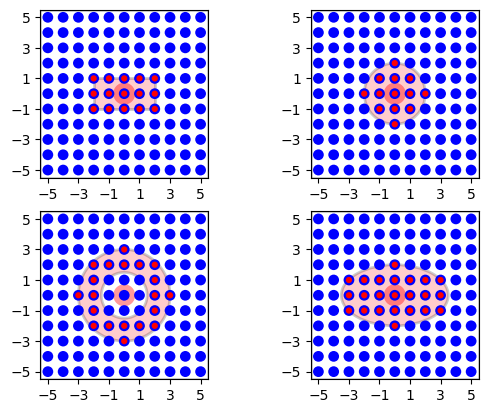Figure 17 Masks for 2D layers. For all mask types, the driver node is marked by a wide light-red circle, the selected pool nodes by red dots and the masks are red. From left to right, top to bottom: rectangular, circular, doughnut and elliptical masks centered about the driver node.

By default, the masks are centered about the position of the driver node, mapped into the pool layer. You can change the location of the mask relative to the driver node by specifying an 'anchor' entry in the mask dictionary. The anchor is a 2D vector specifying the location of the mask center relative to the driver node, as in the following examples (cf. Figure 18).

conndict = {'rule': 'pairwise_bernoulli',
'p': 1.0,
'mask': {'rectangular': {'lower_left': [-2., -1.],
'upper_right': [2., 1.]},
'anchor': [-1.5, -1.5]}}

conndict = {'rule': 'pairwise_bernoulli',
'p': 1.0,
'mask': {'circular': {'radius': 2.0},
'anchor': [-2.0, 0.0]}}

conndict = {'rule': 'pairwise_bernoulli',
'p': 1.0,
'mask': {'doughnut': {'inner_radius': 1.5,
'outer_radius': 3.},
'anchor': [1.5, 1.5]}}

conndict = {'rule': 'pairwise_bernoulli',
'p': 1.0,
'mask': {'elliptical': {'major_axis': 7.,
'minor_axis': 4.},
'anchor': [2.0, -1.0]}}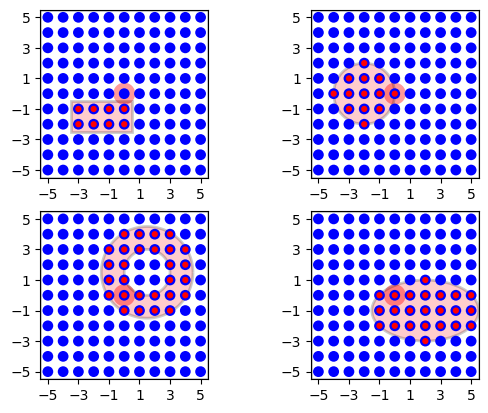Figure 18 The same masks as in Figure 17, but centered about $$(-1.5,-1.5)$$, $$(-2,0)$$, $$(1.5,1.5)$$ and $$(2, -1)$$, respectively, using the 'anchor' parameter.

It is, as of NEST 2.16, possible to rotate the $$\textbf{rectangular}$$ and $$\textbf{elliptical}$$ masks, see Fig Figure 18. To do so, add an 'azimuth_angle' entry in the specific mask dictionary. The azimuth_angle is measured in degrees and is the rotational angle from the x-axis to the y-axis.

conndict = {'rule': 'pairwise_bernoulli',
'p': 1.0,
'mask': {'rectangular': {'lower_left': [-2., -1.],
'upper_right': [2., 1.],
'azimuth_angle': 120.}}}

conndict = {'rule': 'pairwise_bernoulli',
'p': 1.0,
'mask': {'elliptical': {'major_axis': 7.,
'minor_axis': 4.,
'azimuth_angle': 45.}}}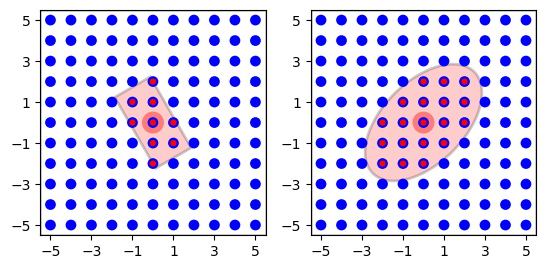#### Masks for 3D layers¶

Similarly, there are three mask types that can be used for 3D NodeCollections,

Box

All nodes within a cuboid volume are connected. The area is specified by its lower left and upper right corners, measured in the same unit as element coordinates. Example:

conndict = {'rule': 'pairwise_bernoulli',
'p': 1.0,
'mask': {'box': {'lower_left': [-2., -1., -1.],
'upper_right': [2., 1., 1.]}}}

Spherical

All nodes within a sphere are connected. The area is specified by its radius.

conndict = {'rule': 'pairwise_bernoulli',
'p': 1.0,
'mask': {'spherical': {'radius': 2.5}}}

Ellipsoidal

All nodes within an ellipsoid are connected. The area is specified by its major, minor, and polar axis.

conndict = {'rule': 'pairwise_bernoulli',
'p': 1.0,
'mask': {'ellipsoidal': {'major_axis': 7.,
'minor_axis': 4.,
'polar_axis': 4.5}}}


As in the 2D case, you can change the location of the mask relative to the driver node by specifying a 3D vector in the 'anchor' entry in the mask dictionary. If you want to rotate the box or ellipsoidal masks, you can add an 'azimuth_angle' entry in the specific mask dictionary for rotation from the x-axis towards the y-axis about the z-axis, or a 'polar_angle' entry, specifying the rotation angle in degrees from the z-axis about the (possibly rotated) x axis, from the (possibly rotated) y-axis. You can specify both at once of course. If both are specified, we first rotate about the z-axis and then about the new x-axis. NEST currently does not support rotation in all three directions, the rotation from the y-axis about the (possibly rotated) z-axis, from the (possibly rotated) x-axis is missing.Figure 19 Masks for 3D NodeCollections. For all mask types, the driver node is marked by a wide light-red circle, the selected pool nodes by red dots and the masks are red. From left to right: box and spherical masks centered about the driver node.

#### Masks for grid-based layers¶

Grid-based layers can be connected using rectangular grid masks. For these, you specify the size of the mask not by lower left and upper right corner coordinates, but give their size in x and y direction, as in this example:

conndict = {'rule': 'pairwise_bernoulli',
'p': 1.0,
'mask': {'grid': {'shape': [5, 3]}}}


The resulting connections are shown in Figure 20. By default the top-left corner of a grid mask, i.e., the grid mask element with grid index $$[0,0]$$ 2, is aligned with the driver node. You can change this alignment by specifying an anchor for the mask:

conndict = {'rule': 'pairwise_bernoulli',
'p': 1.0,
'mask': {'grid': {'shape': [5, 3]},
'anchor': [2, 1]}}


You can even place the anchor outside the mask:

conndict = {'rule': 'pairwise_bernoulli',
'p': 1.0,
'mask': {'grid': {'shape': [3, 5]},
'anchor': [2, -1]}}


The resulting connection patterns are shown in Figure 20.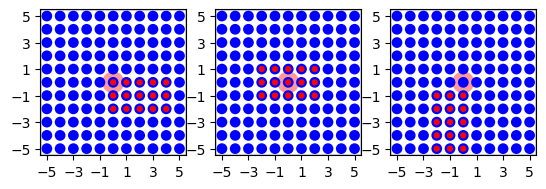Figure 20 Grid masks for connections between grid-based layers. Left: $$5\times 3$$ mask with default alignment at upper left corner. Center: Same mask, but anchored to center node at grid index $$[1,2]$$. Right: Same mask, but anchor to the upper left of the mask at grid index $$[-1,2]$$.

Connections specified using grid masks are generated more efficiently than connections specified using other mask types.

Note the following:

• Grid-based masks are applied by considering grid indices. The position of nodes in physical coordinates is ignored.

• In consequence, grid-based masks should only be used between NodeCollections with identical grid spacings.

• The semantics of the 'anchor' property for grid-based masks differ significantly for general masks described in Sec. 3.3.1. For general masks, the anchor is the center of the mask relative to the driver node. For grid-based nodes, the anchor determines which mask element is aligned with the driver element.

### Probabilistic connection rules¶

Many neuronal network models employ probabilistic connection rules. NEST supports probabilistic connections through the pairwise_bernoulli connection rule. The probability can then be a constant, depend on the position of the source or the target neuron, or on the distance between a driver and a pool node to a connection probability. To create dependencies on neuron positions, NEST parameters objects are used. NEST then generates a connection according to this probability.

Probabilistic connections between layers can be generated in two different ways:

Free probabilistic connections using pairwise_bernoulli

In this case, Connect considers each driver node $$D$$ in turn. For each $$D$$, it evaluates the parameter value for each pool node $$P$$ within the mask and creates a connection according to the resulting probability. This means in particular that each possible driver-pool pair is inspected exactly once and that there will be at most one connection between each driver-pool pair.

Prescribed number of connections

can be obtained by using fixed_indegree or fixed_outdegree connection rule, and specifying the number of connections to create per driver node. See Sec. 3.7 for details.

A selection of specific NEST parameters pertaining to spatially structured networks are shown in Table Spatially-structured specific NEST parameters.

#### Spatially-structured specific NEST parameters¶

The parameters in the table below represent positions of neurons or distances between two neurons. To set node parameters, only the node position can be used. The others can only be used when connecting.

Parameter

Description

nest.spatial.pos.x
nest.spatial.pos.y
nest.spatial.pos.z
Position of a neuron, on the x, y, and z axis.
Can be used to set node properties, but not for connecting.
nest.spatial.source_pos.x
nest.spatial.source_pos.y
nest.spatial.source_pos.z
Position of the source neuron, on the x, y, and z axis.
Can only be used when connecting.
nest.spatial.target_pos.x
nest.spatial.target_pos.y
nest.spatial.target_pos.z
Position of the target neuron, on the x, y, and z axis.
Can only be used when connecting.
nest.spatial.distance
Distance between two nodes. Can only be used when connecting.
nest.spatial.distance.x
nest.spatial.distance.y
nest.spatial.distance.z
Distance on the x, y and z axis between the source and target neuron.
Can only be used when connecting.

NEST provides some functions to help create distributions based on the position of the nodes, for instance the distance between two neurons, shown in the table below. The table also includes parameters drawing values from random distributions.

Distribution function

Arguments

Function

nest.spatial_distributions.exponential()

x,
beta
$p(x) = e^{-\frac{x}{\beta}}$

nest.spatial_distributions.gaussian()

x,
mean,
std
$p(x) = e^{-\frac{(x-\text{mean})^2} {2\text{std}^2}}$

nest.spatial_distributions.gaussian2D()

x,
y,
mean_x,
mean_y,
std_x,
std_y,
rho
$p(x) = e^{-\frac{\frac{(x-\text{mean_x})^2} {\text{std_x}^2}+\frac{ (y-\text{mean_y})^2}{\text{std_y}^2}+2 \rho\frac{(x-\text{mean_x})(y-\text{mean_y})} {\text{std_x}\text{std_y}}} {2(1-\rho^2)}}$

nest.spatial_distributions.gamma()

x,
kappa
$p(x) = \frac{x^{\kappa-1}e^{-\frac{x} {\theta}}}{\theta^\kappa\Gamma(\kappa)}$

nest.random.uniform()

min,
max

$$p\in [\text{min},\text{max})$$ uniformly

nest.random.normal()

mean,
std

normal with given mean and standard deviation

nest.random.exponential()

beta

exponential with a given scale, $$\beta$$

nest.random.lognormal()

mean,
std

lognormal with given mean and standard deviation, std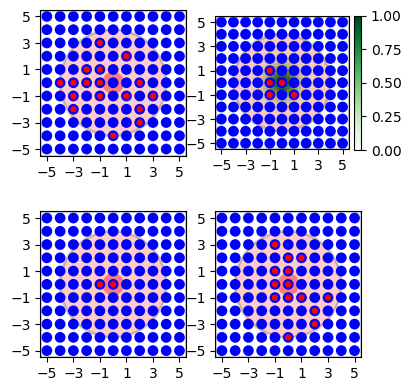Figure 21 Illustration of various connection probabilities. Top left: constant probability, $$p=0.5$$. Top right: Distance dependent Gaussian probability, green distribution show $$\sigma$$. Bottom left: Same distance dependent Gaussian probability, but all $$p<0.5$$ treated as $$p=0$$. Bottom right: 2D-Gaussian.

Several examples follow. They are illustrated in Figure 21.

Constant

Fixed connection probability:

conndict = {'rule': 'pairwise_bernoulli',
'p': 0.5,
'mask': {'circular': {'radius': 4.}}}

Gaussian

The connection probability is a Gaussian distribution based on the distance between neurons. In the example, connection probability is 1 for $$d=0$$ and falls off with a “standard deviation” of $$\sigma=1$$:

conndict = {'rule': 'pairwise_bernoulli',
'p': nest.spatial_distributions.gaussian(nest.spatial.distance, std=1.0),
'mask': {'circular': {'radius': 4.}}}

Cut-off Gaussian

In this example we have a distance-dependent Gaussian distributon, where all probabilities less than $$0.5$$ are set to zero:

distribution = nest.spatial_distributions.gaussian(nest.spatial.distance, std=1.0)
conndict = {'rule': 'pairwise_bernoulli',
'p': nest.logic.conditional(distribution > 0.5,
distribution,
0),
'mask': {'circular': {'radius': 4.}}}

2D Gaussian

We conclude with an example using a two-dimensional Gaussian distribution, i.e., a Gaussian with different widths in $$x$$- and $$y$$- directions. This probability depends on displacement, not only on distance:

conndict = {'rule': 'pairwise_bernoulli',
'p': nest.spatial_distributions.gaussian2D(nest.spatial.distance.x,
nest.spatial.distance.y,
std_x=1.,
std_y=3.),
'mask': {'circular': {'radius': 4.}}}


Note that for pool layers with periodic boundary conditions, NEST always uses the shortest possible displacement vector from driver to pool neuron as nest.spatial.distance.

### Weights and delays¶

Parameters, such as those presented in Table Spatially-structured specific NEST parameters, can also be used to specify distance-dependent or randomized weights and delays for the connections created by Connect. Weight and delays are in NEST passed along in a synapse dictionary to the Connect call.

nest.Connect(nodes, nodes, conn_dict, syn_dict)


Figure Figure 22 illustrates weights and delays generated using these parameters. The code examples used to generate the figures are shown below. All examples use a spatially distributed NodeCollection of 51 nodes placed on a line; the line is centered about $$(25,0)$$, so that the leftmost node has coordinates $$(0,0)$$. The distance between neighboring elements is 1. The mask is rectangular, spans the entire NodeCollection and is centered about the driver node.

Linear example
pos = nest.spatial.grid(shape=[51, 1], extent=[51., 1.], center=[25., 0.])
spatial_nodes = nest.Create('iaf_psc_alpha', positions=pos)

cdict = {'rule': 'pairwise_bernoulli',
'p': 1.0,
'mask': {'rectangular': {'lower_left': [-25.5, -0.5],
'upper_right': [25.5, 0.5]}}}
sdict = {'weight': nest.math.max(1.0 - 0.05 * nest.spatial.distance, 0.),
'delay': 0.1 + 0.02 * nest.spatial.distance}

nest.Connect(spatial_nodes, spatial_nodes, cdict, sdict)


Results are shown in the top panel of Figure 22. Connection weights and delays are shown for the leftmost neuron as driver. Weights drop linearly from $$1$$. From the node at $$(20,0)$$ on, the cutoff sets weights to 0. There are no connections to nodes beyond $$(25,0)$$, since the mask extends only 25 units to the right of the driver. Delays increase in a stepwise linear fashion, as NEST requires delays to be multiples of the simulation resolution.

Linear example with periodic boundary conditions
cdict = {'rule': 'pairwise_bernoulli',
'p': 1.0,
'mask': {'rectangular': {'lower_left': [-25.5, -0.5],
'upper_right': [25.5, 0.5]}}}
sdict = {'weight': nest.math.max(1.0 - 0.05 * nest.spatial.distance, 0.),
'delay': 0.1 + 0.02 * nest.spatial.distance}


Results are shown in the middle panel of Figure 22. This example is identical to the previous, except that the (pool) layer has periodic boundary conditions. Therefore, the left half of the mask about the node at $$(0,0)$$ wraps back to the right half of the layer and that node connects to all nodes in the layer.

Various spatially dependent distributions
cdict = {'rule': 'pairwise_bernoulli',
'p': 1.0,
'mask': {'rectangular': {'lower_left': [-25.5, -0.5],
'upper_right': [25.5, 0.5]}}}
sdict = {'weight': nest.spatial_distributions.exponential(nest.spatial.distance, beta=5.)}

cdict = {'rule': 'pairwise_bernoulli',
'p': 1.0,
'mask': {'rectangular': {'lower_left': [-25.5, -0.5],
'upper_right': [25.5, 0.5]}}}
sdict = {'weight': nest.spatial_distributions.gaussian(nest.spatial.distance, std=5.)}


Results are shown in the bottom panel of Figure 22. It shows linear, exponential and Gaussian distributions of the distance between connected nodes, used with weight functions for the node at $$(25,0)$$.

Randomized weights and delays
cdict = {'rule': 'pairwise_bernoulli',
'p': 1.0,
'mask': {'rectangular': {'lower_left': [-25.5, -0.5],
'upper_right': [25.5, 0.5]}}}
sdict = {'weight': nest.random.uniform(min=0.2, max=0.8)}


By using the nest.random.uniform() parameter for weights or delays, one can obtain randomized values for weights and delays, as shown by the red circles in the bottom panel of Figure 22.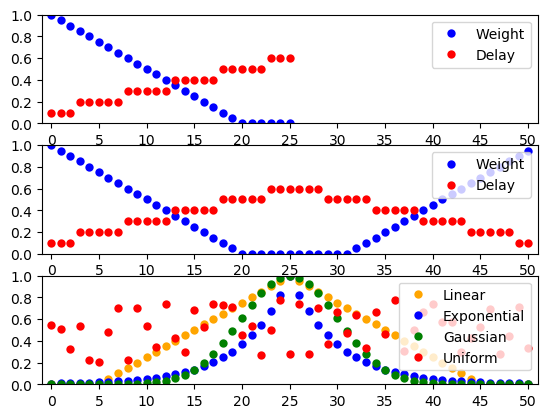Figure 22 Distance-dependent and randomized weights and delays. See text for details.

### Designing distance-dependent parameters¶

Although NEST comes with some pre-defined functions that can be used to create distributions of distance-dependent parameters, there is no limit to how parameters can be combined.

As an example, we will now combine some parameters to create a new parameter that is linear (actually affine) with respect to the displacement between the nodes, of the form

$p = 0.5 + d_x + 2 d_y.$

where $$d_x$$ and $$d_y$$ are the displacements between the source and target neuron on the x and y axis, respectively. The parameter is then simply:

parameter = 0.5 + nest.spatial.distance.x + 2. * nest.spatial.distance.y


This can be directly plugged into the Connect function:

spatial_nodes = nest.Create('iaf_psc_alpha',
positions=nest.spatial.grid(shape=[11, 11],
extent=[1., 1.]))
nest.Connect(spatial_nodes, spatial_nodes, {'rule': 'pairwise_bernoulli',
'p': parameter,
'mask': {'circular': {'radius': 0.5}}})


### Periodic boundary conditions¶

Connections between layers with periodic boundary conditions are based on the following principles:

• Periodic boundary conditions are always applied in the pool layer. It is irrelevant whether the driver layer has periodic boundary conditions or not.

• By default, NEST does not accept masks that are wider than the pool layer when using periodic boundary conditions. Otherwise, one pool node could appear as multiple targets to the same driver node as the masks wraps several times around the layer. For layers with different extents in $$x$$- and $$y$$-directions this means that the maximum layer size is determined by the smaller extension.

• nest.spatial.distance and its single dimension variants always consider the shortest distance (displacement) between driver and pool node.

In most physical systems simulated using periodic boundary conditions, interactions between entities are short-range. Periodic boundary conditions are well-defined in such cases. In neuronal network models with long-range interactions, periodic boundary conditions may not make sense. In general, we recommend to use periodic boundary conditions only when connection masks are significantly smaller than the NodeCollections they are applied to.

### Prescribed number of connections¶

We have so far described how to connect spatially distributed NodeCollections by either connecting to all nodes inside the mask or by considering each pool node in turn and connecting it according to a given probability function. In both cases, the number of connections generated depends on mask and connection probability.

Many neuron models in the literature, in contrast, prescribe a certain fan in (number of incoming connections) or fan out (number of outgoing connections) for each node. You can achieve this in NEST by prescribing the number of connections for each driver node by using fixed_indegree or fixed_outdegree as connection rule.

Connection generation now proceeds in a different way than before:

1. For each driver node, Connect randomly selects a node from the mask region in the pool layer, and creates a connection with the probability prescribed. This is repeated until the requested number of connections has been created.

2. Thus, if all nodes in the mask shall be connected with equal probability, you should not specify a connection probability.

3. If you specify a probability with a distance-dependent distribution (e.g., Gaussian, linear, exponential), the connections will be distributed within the mask with the spatial profile given by the probability.

4. If you prohibit multapses (cf Sec. 3.1.1) and prescribe a number of connections greater than the number of pool nodes in the mask, Connect may get stuck in an infinite loop and NEST will hang. Keep in mind that the number of nodes within the mask may vary considerably for free layers with randomly placed nodes.

5. If you use the connection rule 'rule': fixed_indegree in the connection dictionary, you also have to specify 'indegree', the number of connections per target node.

6. Similarly, if you use the connection rule 'rule': fixed_outdegree in the connection dictionary, you have to use 'outdegree' to specify the number of connections per source node.

The following code generates a network of 1000 randomly placed nodes and connects them with a fixed fan out, of 50 outgoing connections per node distributed with a profile linearly decaying from unit probability to zero probability at distance $$0.5$$. Multiple connections (multapses) between pairs of nodes are allowed, self-connections (autapses) prohibited. The probability of finding a connection at a certain distance is then given by the product of the probabilities for finding nodes at a certain distance with the probability value for this distance. For the connection probability and parameter values below we have

$p_{\text{conn}}(d) = \frac{12}{\pi} \times 2\pi r \times (1-2r) = 24 r (1-2r) \qquad \text{for} \quad 0\le r < \frac{1}{2}\;.\qquad$
The resulting distribution of distances between connected nodes is shown in
pos = nest.spatial.free(nest.random.uniform(-1., 1.),
extent=[2., 2.], edge_wrap=True)
spatial_nodes = nest.Create('iaf_psc_alpha', 1000, positions=pos)

cdict = {'rule': 'fixed_outdegree',
'p': nest.math.max(1. - 2 * nest.spatial.distance, 0.),
'mask': {'circular': {'radius': 1.0}},
'outdegree': 50,
'allow_multapses': True, 'allow_autapses': False}
nest.Connect(spatial_nodes, spatial_nodes, cdict)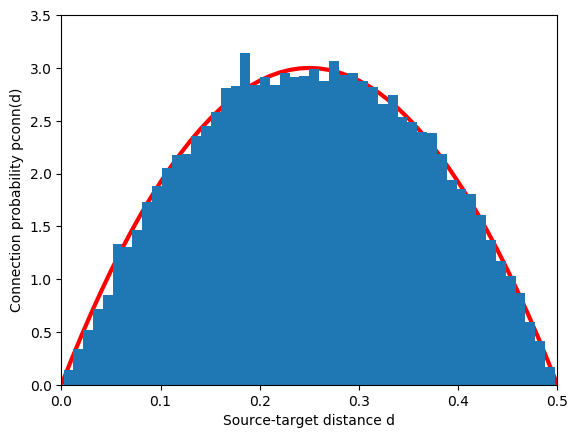Figure 23 Distribution of distances between source and target for a network of 1000 randomly placed nodes, a fixed fan out of 50 connections and a connection probability decaying linearly from 1 to 0 at $$d=0.5$$. The red line is the expected distribution from Eq. eq_ptheo.

Functions determining weight and delay as function of distance/displacement work in just the same way as before when the number of connections is prescribed.

### Synapse models and properties¶

By default, Connect creates connections using the default synapse model in NEST, static_synapse. You can specify a different model by adding a 'synapse_model' entry to the synapse specification dictionary, as in this example:

nest.ResetKernel()
nest.CopyModel('static_synapse', 'exc', {'weight': 2.0})
nest.CopyModel('static_synapse', 'inh', {'weight': -8.0})

pos = nest.spatial.grid(shape=[10, 10])
ex_nodes = nest.Create('iaf_psc_alpha', positions=pos)
in_nodes = nest.Create('iaf_psc_alpha', positions=pos)

nest.Connect(ex_nodes, in_nodes, {'rule': 'pairwise_bernoulli',
'p': 0.8,
'mask': {'circular': {'radius': 0.5}}},
{'synapse_model': 'exc'})
nest.Connect(in_nodes, ex_nodes, {'rule': 'pairwise_bernoulli',
'p': 1.0,
'mask': {'rectangular': {'lower_left': [-0.2, -0.2],
'upper_right': [0.2, 0.2]}}},
{'synapse_model': 'inh'})


You have to use synapse models if you want to set, e.g., the receptor type of connections or parameters for plastic synapse models. These can not be set in distance-dependent ways at present.

### Connecting devices to subregions of NodeCollections¶

It is possible to connect stimulation and recording devices only to specific subregions of the layers. A simple way to achieve this is to create a layer which contains only the device placed typically in its center. When connecting the device layer to a neuron layer, an appropriate mask needs to be specified and optionally also an anchor for shifting the center of the mask. As demonstrated in the following example, stimulation devices have to be connected as the source layer.

nrn_layer = nest.Create('iaf_psc_alpha',
positions=nest.spatial.grid(shape=[20, 20]))

stim = nest.Create('poisson_generator',
positions=nest.spatial.grid(shape=[1, 1]))

cdict_stim = {'rule': 'pairwise_bernoulli',
'p': 1.0,
'mask': {'circular': {'radius': 0.1},
'anchor': [0.2, 0.2]}}

nest.Connect(stim, nrn_layer, cdict_stim)


While recording devices, on the other hand, have to be connected as the target layer (see also Sec. 3.11):

rec = nest.Create('spike_recorder',
positions=nest.spatial.grid(shape=[1, 1]))

cdict_rec = {'rule': 'pairwise_bernoulli',
'p': 1.0,
'use_on_source': True,
'mask': {'circular': {'radius': 0.1},
'anchor': [-0.2, 0.2]}}

nest.Connect(nrn_layer, rec, cdict_rec)


### Spatially distributed NodeCollections and recording devices¶

Generally, one should not create a layer of recording devices to record from another NodeCollection with spatial extent. This is especially true for spike recorders. Instead, create a single spike recorder and connect all neurons in the spatially distributed NodeCollection to that spike recorder:

rec = nest.Create('spike_recorder')
nest.Connect(nrn_layer, rec)


Connecting a layer of neurons to a layer of recording devices as described in Sec. 3.10, is only possible using the pairwise_bernoulli rule. Note that voltmeter and multimeter do not suffer from this restriction, since they are connected as sources, not as targets.

## Inspecting Spatially distributed NodeCollections¶

We strongly recommend that you inspect the NodeConnections created to be sure that node placement and connectivity indeed turned out as expected. In this chapter, we describe some functions that NEST provide to query and visualize networks, NodeCollections, and connectivity.

### Query functions¶

The following table presents some query functions provided by NEST.

 nest.PrintNodes() Print the node ID ranges and model names of the nodes in the network. nest.GetConnections() Retrieve connections (all or for a given source or target); see also http://www.nest-simulator.org/connection_management. nest.GetNodes() Returns a NodeCollection of all elements with given properties. nest.GetPosition() Return the spatial locations of nodes. nest.GetTargetNodes() Obtain targets of sources in a given target layer. nest.GetTargetPositions() Obtain positions of targets of sources in a given target layer. nest.FindNearestElement() Return the node(s) closest to the location(s) in the given NodeCollection. nest.FindCenterElement() Return NodeCollection of node closest to center of layer. nest.Displacement() Obtain vector of lateral displacement between nodes, taking periodic boundary conditions into account. nest.Distance() Obtain vector of lateral distances between nodes, taking periodic boundary conditions into account. nest.DumpLayerNodes() Write layer element positions to file. nest.DumpLayerConnections() Write connectivity information to file. This function may be very useful to check that NEST created the correct connection structure. nest.SelectNodesByMask() Obtain NodeCollection of elements inside a masked area of a NodeCollection.

### Visualization functions¶

NEST provides three functions to visualize networks:

 PlotLayer() Plot nodes in a spatially distributed NodeCollection. PlotTargets() Plot all targets of a node in a given NodeCollection. PlotProbabilityParameter() Add indication of mask and probability p to plot of NodeCollection. This function is usually called by PlotTargets.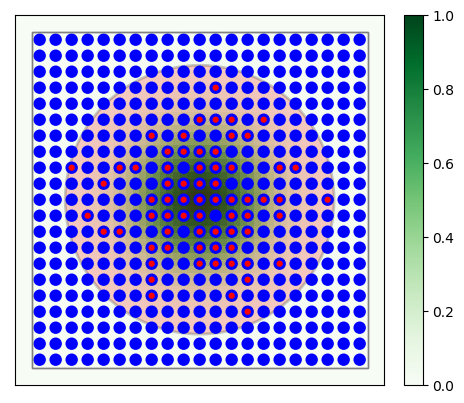Figure 24 $$21\times 21$$ grid with divergent Gaussian projections onto itself. Blue circles mark layer elements, red circles connection targets of the center neuron. The large red circle is the mask, the green distribution mark the Gaussian probability distribution.

The following code shows a practical example: A $$21\times21$$ network which connects to itself with Gaussian connections. The resulting graphics is shown in Figure 24. All elements and the targets of the center neuron are shown, as well as mask and connection probability.

layer = nest.Create('iaf_psc_alpha',
positions=nest.spatial.grid(shape=[21, 21]))
probability_param = nest.spatial_distributions.gaussian(nest.spatial.distance, std=0.15)
conndict = {'rule': 'pairwise_bernoulli',
'p': probability_param,
'mask': {'circular': {'radius': 0.4}}}
nest.Connect(layer, layer, conndict)
fig = nest.PlotLayer(layer, nodesize=80)

ctr = nest.FindCenterElement(layer)
nest.PlotTargets(ctr, layer, fig=fig,
mask=conndict['mask'], probability_parameter=probability_param,
src_size=250, tgt_color='red', tgt_size=20, mask_color='red',
probability_cmap='Greens')


## Creating custom masks¶

In some cases, the built-in masks may not meet your needs, and you want to create a custom mask. There are two ways to do this:

1. To use parameters to introduce a cut-off to the connection probability.

2. To implement a custom mask in C++ as a module.

Using parameters is the most accessible option; the entire implementation is done on the PyNEST level. However, the price for this flexibility is reduced connection efficiency compared to masks implemented in C++. Combining parameters to give the wanted behaviour may also be difficult if the mask specifications are complex.

Implementing a custom mask in C++ gives much higher connection performance and greater freedom in implementation, but requires some knowledge of the C++ language. As the mask in this case is implemented in an extension module, which is dynamically loaded into NEST, it also requires some additional steps for installation.

### Using parameters to specify connection boundaries¶

You can use parameters that represent spatial distances between nodes to create a connection probability with mask behaviour. For this, you need to create a condition parameter that describes the boundary of the mask. As condition parameters evaluate to either 0 or 1, it can be used alone, with the nest.logic.conditional() parameter, or multiplied with another parameter or value, before passing it as the connection probability.

As an example, suppose we want to create connections to 50 % of the target nodes, but only to those within an elliptical area around each source node. Using nest.spatial.distance parameters, we can define a parameter that creates an elliptical connection boundary.

First, we define variables controlling the shape of the ellipse.

rx = 0.5   # radius in the x-direction
ry = 0.25  # radius in the y-direction


Next, we define the connection boundary. We only want to connect to targets inside an ellipse, so the condition is

$\frac{x^2}{r_x^2}+\frac{y^2}{r_y^2} \leq 1,$

where $$x$$ and $$y$$ are the distances between the source and target neuron, in x- and y-directions, respectively. We use this expression to define the boundary using parameters.

x = nest.spatial.distance.x
y = nest.spatial.distance.y
lhs = x * x / rx**2 + y * y / ry**2
mask_param = nest.logic.conditional(lhs <= 1.0, 0.5, 0.0)
# Because the probability outside the ellipse is zero,
# we could also have defined the parameter as
# mask_param = 0.5*(lhs <= 1.0)


Then, we can use the parameter as connection probability when connecting populations with spatial information.

l = nest.Create('iaf_psc_alpha', positions=nest.spatial.grid(shape=[11, 11], extent=[1., 1.]))
nest.Connect(l, l, {'rule': 'pairwise_bernoulli', 'p': mask_param})


### Adding masks in a module¶

If using parameters to define a connection boundary is not efficient enough, or if you need more flexibility in defining the mask, you can add a custom mask, written in C++, and add it to NEST via an extension module. For more information on writing such modules, see the NEST extension module repository.

To add a mask, a subclass of nest::Mask<D> must be defined, where D is the dimension (2 or 3). In this case we will define a 2-dimensional elliptic mask by creating a class called EllipticMask. Note that elliptical masks are already part of NEST see Sec. 3.3. That elliptical mask is defined in a different way than what we will do here though, so this can still be used as an introductory example. First, we must include the header files for the Mask parent class:

#include "mask.h"
#include "mask_impl.h"


The Mask class has a few methods that must be overridden:

class EllipticMask : public nest::Mask< 2 >
{
public:
EllipticMask( const DictionaryDatum& d )
: rx_( 1.0 )
, ry_( 1.0 )
{
updateValue< double >( d, "r_x", rx_ );
updateValue< double >( d, "r_y", ry_ );
}

using Mask< 2 >::inside;

// returns true if point is inside the ellipse
bool
inside( const nest::Position< 2 >& p ) const
{
return p[ 0 ] * p[ 0 ] / rx_ / rx_ + p[ 1 ] * p[ 1 ] / ry_ / ry_ <= 1.0;
}

// returns true if the whole box is inside the ellipse
bool
inside( const nest::Box< 2 >& b ) const
{
nest::Position< 2 > p = b.lower_left;

// Test if all corners are inside mask
if ( not inside( p ) )
return false; // (0,0)
p[ 0 ] = b.upper_right[ 0 ];
if ( not inside( p ) )
return false; // (0,1)
p[ 1 ] = b.upper_right[ 1 ];
if ( not inside( p ) )
return false; // (1,1)
p[ 0 ] = b.lower_left[ 0 ];
if ( not inside( p ) )
return false; // (1,0)

return true;
}

// returns bounding box of ellipse
nest::Box< 2 >
get_bbox() const
{
nest::Position< 2 > ll( -rx_, -ry_ );
nest::Position< 2 > ur( rx_, ry_ );
return nest::Box< 2 >( ll, ur );
}

nest::Mask< 2 >*
clone() const
{
return new EllipticMask( *this );
}

protected:
double rx_, ry_;
};


The overridden methods include a test if a point is inside the mask, and for efficiency reasons also a test if a box is fully inside the mask. We implement the latter by testing if all the corners are inside, since our elliptic mask is convex. We must also define a function which returns a bounding box for the mask, i.e. a box completely surrounding the mask.

The mask class must then be registered in NEST. This is done by adding a line to the function MyModule::init() in the file mymodule.cpp:

nest::NestModule::register_mask< EllipticMask >( "elliptic" );


After compiling and installing your module, the mask is available to be used in connections, e.g.

nest.Install('mymodule')
l = nest.Create('iaf_psc_alpha', positions=nest.spatial.grid(shape=[11, 11], extent=[1., 1.]))
nest.Connect(l, l, {'rule': 'pairwise_bernoulli',
'p': 0.5,
'mask': {'elliptic': {'r_x': 0.5, 'r_y': 0.25}}})